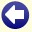## Solution to: Word Sums

It is given that the digits 1 and 6 are the most frequently used, so we first look how often each letter occurs in the puzzle:

• M, V, and P occur once;
• T occurs twice;
• A, R, and E occur three times;
• S occurs four times;
• N and U occur five times.

So, either N=1 and U=6, or N=6 and U=1. Since even 9999 + 99999 + 999999 + 999999 is less than 6000000, N cannot be 6. So N=1 and U=6.

We now have:

```     mars
ve16s
6ra16s
sat6r1
------- +
1ept61e
```

R+6+6+R (in the 'tens' column) is even, but since its sum ends on a 1, 1 must have been carried from the 'units' column. We can also see that R=4 or R=9. The fact that 1 is carried from the 'units' to the 'tens' column gives the following possible values for S:

• S=3, so S+S+S+1=10, and therefore E=0;
• S=4, so S+S+S+1=13, and therefore E=3;
• S=5, so S+S+S+1=16, and therefore E=6: this is not possible because U=6.

Depending on the values of S and E, we now have:

```S=3 and E=0:    S=4 and E=3:
mar3            mar4
v0163           v3164
6ra163          6ra164
3at6r1          4at6r1
------- +       ------- +
10pt610         13pt613
```

From the previous step, we know that R=4 or R=9. We first look at the case S=3. If R would be 4, a value of 2 would be carried from the 'tens' to the 'hundreds' column. So, the sum of 2+A+1+1+6 must end on a 6, which gives A=6. This is not possible because U=6. So, if S=3, then R must be 9 and a value of 3 is carried from the 'tens' to the 'hundreds' column. So, the sum of 3+A+1+1+6 must end on a 6, which gives A=5.

We now look at the case S=4. In this case, R can only be 9. Then a value of 3 is carried from the 'tens' to the 'hundreds' column, which gives A=5.

Therefore, regardless of the values of S and E, we know that R=9 and A=5. Depending on the values of S and E, we now have:

```S=3 and E=0:    S=4 and E=3:
m593            m594
v0163           v3164
695163          695164
35t691          45t691
------- +       ------- +
10pt610         13pt613
```

In either case, a value of 1 is carried from the 'hundreds' to the 'thousands' column. In the case that S=3 and E=0, the sum of 1+M+0+5+T must end on T, which gives M=4. In the case that S=4 and E=3, the sum of 1+M+3+5+T must end on T, which gives M=1. This, however, is not possible because U=1. From this, it follows that S cannot be 4, so we now know that S=3, E=0, and M=4.

We now have:

```     4593
v0163
695163
35t691
------- +
10pt610
```

The only remaining digits are now 2, 7, and 8. Since a value of 1 is carried from the 'thousands' to the 'ten thousands' column, it follows that V=2 and P=7. For T, only the value 8 remains.

The complete addition is as follows:

```     4593
20163
695163
358691
------- +
1078610
```

Conclusion: neptune equals 1078610.Back to the puzzle
This website uses cookies. By further use of this website, or by clicking on 'Continue', you give permission for the use of cookies. If you want more information, look at our cookie policy.